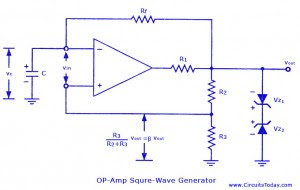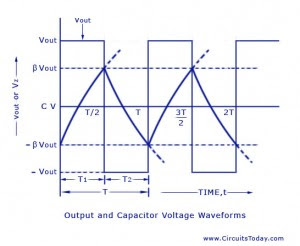## Audio

[Audio][slideshow]

## How to make an Astable or Free running Multi vibrator using 741 Op-Amp ?op amp astable multi vibrator

The non-sinusoidal waveform generators are also called relaxation oscillators. The op-amp relaxation oscillator shown in figure is a square wave generator. In general, square waves are relatively easy to produce. Like the UJT relaxation oscillator, the circuit’s frequency of oscillation is dependent on the charge and discharge of a capacitor C through feedback resistor R,. The “heart” of the oscillator is an inverting op-amp comparator

The compa­rator uses positive feedback that increases the gain of the amplifier. In a comparator circuit this offer two advantages. First, the high gain causes the op-amp’s output to switch very quickly from one state to an­other and vice-versa. Second, the use of positive feedback gives the circuit hysteresis. In the op-amp square-wave generator circuit given in figure, the output voltage vout is shunted to ground by two Zener diodes Z1 and Z2 connected back-to-back and is limited to either VZ 2 or –VZ 1. A fraction of the output is fedback to the non-inverting (+) input terminal. Combination of IL and C acting as a low-pass R-C circuit is used to integrate the output voltage vout and the capacitor voltage vc is applied to the inverting input terminal in place of external signal. The differential input voltage is given as vin = vc – β vout

When vin is positive, vout = – Vz1 and when vin is negative vout = + Vz 2. Consider an instant of time when vin < 0. At this instant vout = + Vz 2 , and the voltage at the non-inverting (+) input terminal is β Vz 2 , the capacitor C charges exponentially towards Vz 2, with a time constant Rf C. The output voltage remains constant at Vz 2 until vc equal β Vz 2.

When it happens, comparator output reverses to – Vz1. Now vc changes exponentially towards -Vz1 with the same time constant and again the output makes a transition from -Vz1 to + Vz 2. when vc equals -βVz 1

Let Vz1 = Vz 2

The time period, T, of the output square wave is determined using the charging and discharging phenomena of the capacitor C. The voltage across the capacitor, vc when it is charging from – B Vz to + Vz is given by

Vc = [1-(1+β)]e-T/2τ

Where τ = RfC

The waveforms of the capacitor voltage vc and output voltage vout (or vz) are shown in figure.

When t = t/2

Vc = +β Vz or + β Vout

Therefore β Vz = Vz [1-(1+β)e-T/2τ]

Or e-T/2τ = 1- β/1+ β

Or T = 2τ loge 1+β/1- β = 2Rf C loge [1+ (2R3/R2)]The frequency, f = 1/T , of the square-wave is independent of output voltage Vout. This circuit is also known as free-running or astable multivibrator because it has two quasi-stable states. The output remains in one state for time T1 and then makes an abrupt transition to the second state and re­mains in that state for time T2. The cycle repeats itself after time T = (T1 + T2) where T is the time period of the square-wave.

The op-amp square-wave generator is useful in the frequency range of about 10 Hz -10 kHz. At higher frequencies, the op-amp’s slew rate limits the slope of the output square wave. The symmetry of the output waveform depends on the matching of two Zener diodes Z1 and Z2. The unsymmetrical square-wave (T1 not equal to t2) can be had by using different constants for charging the capacitor C to +Vout and -Vout

[Lights][grids]